# Factors and Greatest Common Factors

Problem: The area of a rectangular garden is 24 square yards. List all possible whole-number dimensions the garden can have.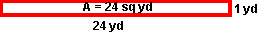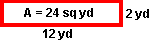Solution: 1 yd x 24 yd,  2 yd x 12 yd,  3 yd x 8 yd,  and 4 yd x 6 yd

The whole-number dimensions of this rectangular garden are the factors of the number 24. The following statements can be made about the factors of 24:

• The factors of 24 are: 1, 2, 3, 4, 6, 8, 12 and 24 since each of these numbers divides exactly into 24.
• The pairs of factors of 24 are: 1 and 24, 2 and 12, 3 and 8, and 4 and 6.

To find the factors of a whole number follow this procedure:

1. Starting with 1, divide each counting number into the whole number.
2. If the numbers divide exactly (no remainder), then you have found a pair of factors.
3. List the counting number and the quotient of your division as a pair of factors.
4. Keep dividing until a factor repeats.
5. List all factors separated by commas.

Example 1: Find the factors of 12.

Counting #   Division Factor Pair
1 12 ÷ 1 = 12 1 x 12
2 12 ÷ 2 = 6 2 x 6
3 12 ÷ 3 = 4 3 x 4
4 12 ÷ 4 = 3 4 x 3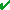Solution: The factors of 12 are 1, 2, 3, 4, 6 and 12.

(Note:means that one or more factors has repeated so we stop dividing.)

Example 2: Find the factors of 20.

Counting # Division Factor Pair
1 20 ÷ 1 = 20 1 x 20
2 20 ÷ 2 = 10 2 x 10
3 20 ÷ 3 = 6 R2 --------
4 20 ÷ 4 = 5 4 x 5
5 20 ÷ 5 = 4 5 x 4Solution: The factors of 20 are 1, 2, 4, 5, 10 and 20.

Example 3: Find the factors of 49.

Counting # Division Factor Pair
1 49 ÷ 1 = 49 1 x 49
2 49 ÷ 2 = 24 R1 --------
3 49 ÷ 3 = 16 R1 --------
4 49 ÷ 4 = 12 R1 --------
5 49 ÷ 5 = 9 R4 --------
6 49 ÷ 6 = 8 R1 --------
7 49 ÷ 7 = 7 7 x 7Solution: The factors of 49 are 1, 7 and 49

Problem: Find the Greatest Common Factor (GCF) of 12 and 20. (Note: Place your mouse over the lists of factors below.)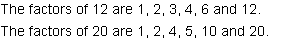The common factors of 12 and 20 are 1, 2 and 4.

The greatest common factor of 12 and 20 is 4.

Solution: GCF = 4

To find the Greatest Common Factor (GCF) of two or more whole numbers, follow this procedure:

1. Make a list of factors for each whole number.
2. Identify all factors that are common to all lists.
3. The Greatest Common Factor (GCF) is the largest of these common factors.

#### In Examples 4 through 6, place your mouse over the lists of factors to see the common factors.

Example 4: Find the GCF of 24 and 36.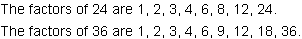The common factors of 24 and 36 are 1, 2, 3, 4, 6 and 12.

The greatest common factor of 24 and 36 is 12.

Solution: GCF = 12

Example 5: Find the GCF of 56 and 63.The common factors of 56 and 63 are 1 and 7.

The greatest common factor of 56 and 63 is 7.

Solution: GCF = 7

Example 6: Find the GCF of 12, 30 and 54.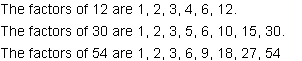The common factors of 12, 30 and 54 are 1, 2, 3 and 6.

The greatest common factor of 12, 30 and 54 is 6.

Solution: GCF = 6

Summary: The factors of a whole number are those numbers that divide exactly into that whole number. The Greatest Common Factor (GCF) of a set of whole numbers is the largest factor common to all whole numbers in the set.

### Exercises

Directions: Read each question below. Click once in an ANSWER BOX and type in your answer; then click ENTER. After you click ENTER, a message will appear in the RESULTS BOX to indicate whether your answer is correct or incorrect. To start over, click CLEAR.

 1 Find the GCF of 18 and 36. ANSWER BOX:  GCF =   RESULTS BOX:
 2 Find the GCF of 30 and 48. ANSWER BOX:  GCF =   RESULTS BOX:
 3 Find the GCF of 42 and 56. ANSWER BOX:  GCF =   RESULTS BOX:
 4 Find the GCF of 16, 48 and 72. ANSWER BOX:  GCF =   RESULTS BOX:
 5 One rectangular garden has an area of 18 square meters. Another rectangular garden has an area of 81 square meters. What is the largest possible whole-number dimension common to both gardens? ANSWER BOX:  GCF =  m  RESULTS BOX: